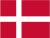### Dish Cloth 49

Cast on 60 stitches and knit 6 rows and then pattern. When the cloth has the desired length, knit 6 rows and bind off.

Pattern:

1.row: 10 p, 2 k
2.row: 2 p, 1 k, 2 p, 1 k, 2 p, 1 k, 2 p, 1 k
3.row: 1 p, 2 k, 1 p, 2 k, 1 p, 2 k, 1 p, 2 k
4.row: 6 k, 2 p, 4 k
5.row: 1 p, 8 k, 1 p, 2 k
6.row: 2 p, 1 k, 8 p, 1 k
7.row: 4 p, 2 k, 6 p
8.row: as row 2
9.row: as row 3
10.row: 2 p, 10 k
11.row: 3 k, 1 p, 2 k, 1 p, 5 k
12.row: 5 p, 1 k, 2 p, 1 k, 3 p

 X X 12 X X 11 X X X X X X X X X X 10 X X X X 9 X X X X 8 X X X X X X X X X X 7 X X 6 X X 5 X X X X X X X X X X 4 X X X X 3 X X X X 2 X X X X X X X X X X 1
You have to remember that all uneven rows start in the right side of the diagram and X=purl and ▢= knit. All equal rows start in the left side of the diagram and X=knit and ▢=purl.Danish